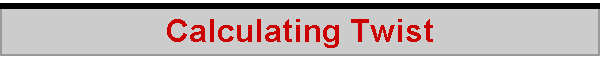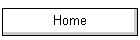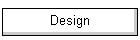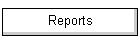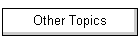One of the little puzzles I've been toying with is that of knowing how much twist gets added to the sail when you ease the kicker a half turn, say, or raise the topping lift by 1 mm.  The downloadable spreadsheet can calculate the amount of twist given these changes, using the formulae shown in the diagrams. To begin with, we make a basic assumption that the leech of the sail is curved in the shape of an arc of a circle. This isn't a wonderful assumption, but it'll do until I find a better one.  As the kicker is eased, or the topping lift shortened, the sail leech gains curvature, and in doing so induces more twist into the sail along its height.  If we know the distance between the leech and the vertical "no twist" position, we can calculate the twist.  To go with our assumption of a circle arc for the leech curve, we also need an approximation in order to calculate the required distance between the leech and the vertical.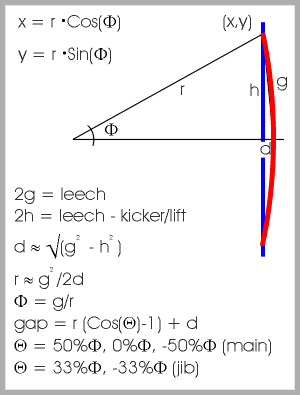The approximation we need, in order to calculate the radius of curvature of the leech, is to say that the leech curve can be approximated by a straight line. This allows us to calculate the gap, "d", in the middle of the leech, and then to estimate the radius of curvature of the leech arc, "r".  The radius of curvature then allows us to calculate the gap between other positions on the leech and the "no twist" position.  We choose to calculate the gaps (and hence the sail twist) at the battens, which are at 25%, 50%, and 75% along the mainsail leech, and at 33% and 67% along the jib leech. The twist is calculated relative to the chord of the sail at the batten of interest.  To simplify things, we assume the sails to be triangular. This doesn't matter, unless you want to go and actually measure the gap to see if you agree with the spreadsheet.  In this case, you just need to remember to measure the gap from the imaginary triangle's hypotenuse on the main and not from the edge of the leech itself... The spreadsheet gives some very interesting results, illustrated in the following graphs.  The clew of the sail only needs to be lifted or eased a small amount to produce all the twist you need.  For the main of an IOM "A" rig, it seems that about 3mm does the trick, giving about 21 degrees of twist at the top batten and 12 degrees at the middle batten.  For the jib, a lift of about 2mm gives approximately 13 degrees twist at the upper batten, and 7 degrees at the lower.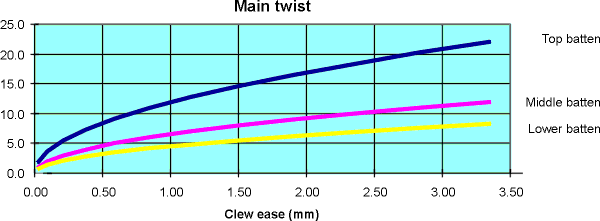For fun, I've taken the calculations from the gooseneck spreadsheet and used them to estimate the number of turns of the kicking strap adjuster screw that gives a change in mainsail twist.  Easing the mainsail clew by 2mm is equivalent to about a one-third turn of the kicker -- an astonishingly small adjustment that gives a correspondingly huge change in twist.  The "full range" of adjustment is around two-thirds of a turn.  I had no idea the kicker -- and twist -- was so sensitive. Another interesting finding from the spreadsheet is a take on the often-heard comment "Adjust your jib so its curve matches that of the main".  One way to interpret the suggestion is to say that the radius of curvature of the jib leech should be similar to the radius of curvature of the mainsail leech, and these radiuses are provided by the spreadsheet.  It turns out that a radius of about 7000mm for the main yields twists of 20, 11, and 7 degrees at its battens, while a radius of 7000mm for the jib yields twists of 10 and 5 degrees.  Given that the jib upper batten usually aligns with the main middle batten, and its lower batten with the main bottom batten, we have quite good agreement.  There may be something in that advice after all...! 2005-12-18